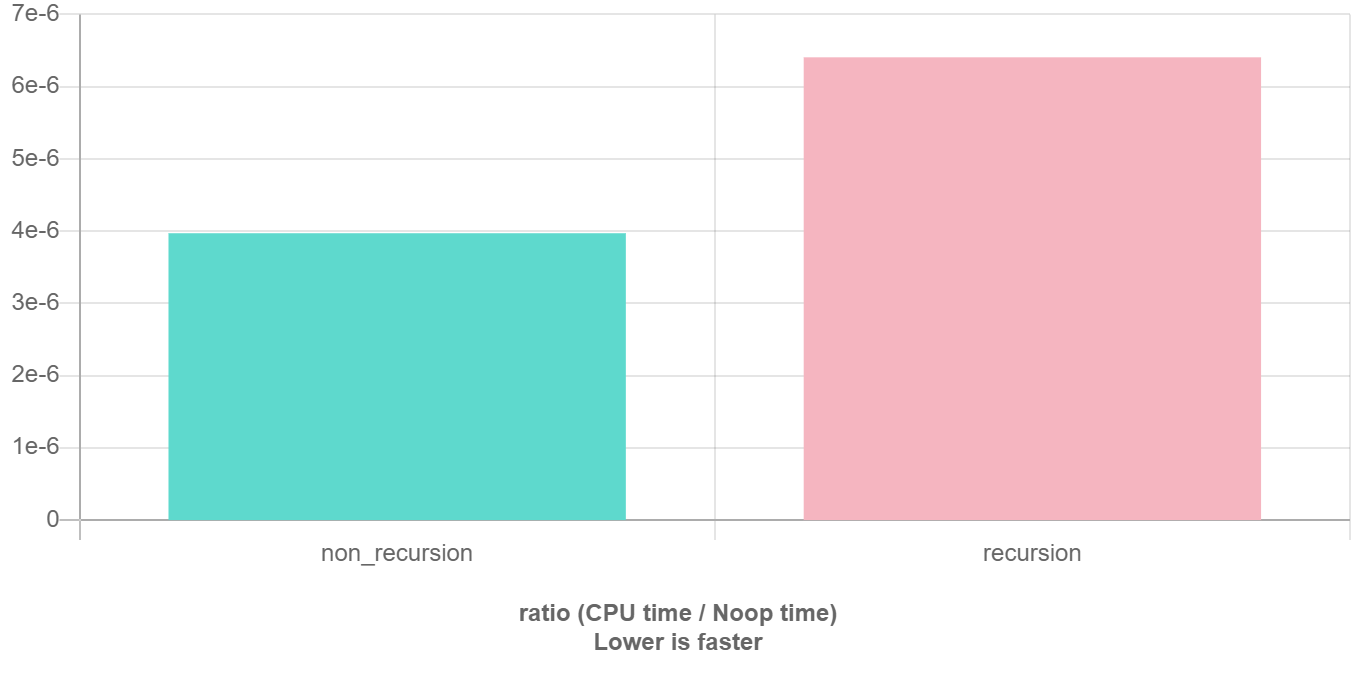# 递归 & 分治

## 详细介绍

### 递归

1. 什么是递归？
2. 如何给一堆数字排序？答：分成两半，先排左半边再排右半边，最后合并就行了，至于怎么排左边和右边，请重新阅读这句话。
3. 你今年几岁？答：去年的岁数加一岁，1999 年我出生。
4.```1 2 3 4``` ``````int func(传入数值) { if (终止条件) return 最小子问题解; return func(缩小规模); } ``````

#### 为什么要写递归

1. 结构清晰，可读性强。例如，分别用不同的方法实现 归并排序

 ``` 1 2 3 4 5 6 7 8 9 10 11 12 13 14 15 16 17 18 19``` ``````// C++ Version // 不使用递归的归并排序算法 template void merge_sort(vector a) { int n = a.size(); for (int seg = 1; seg < n; seg = seg + seg) for (int start = 0; start < n - seg; start += seg + seg) merge(a, start, start + seg - 1, std::min(start + seg + seg - 1, n - 1)); } // 使用递归的归并排序算法 template void merge_sort(vector a, int front, int end) { if (front >= end) return; int mid = front + (end - front) / 2; merge_sort(a, front, mid); merge_sort(a, mid + 1, end); merge(a, front, mid, end); } ``````
 ``` 1 2 3 4 5 6 7 8 9 10 11 12 13 14 15 16 17 18 19``` ``````# Python Version #不使用递归的归并排序算法 def merge_sort(a): n = len(a) seg, start = 1, 0 while seg < n: while start < n - seg: merge(a, start, start + seg - 1, min(start + seg + seg - 1, n - 1)) start = start + seg + seg seg = seg + seg #使用递归的归并排序算法 def merge_sort(a, front, end): if front >= end: return mid = front + (end - front) / 2 merge_sort(a, front, mid) merge_sort(a, mid + 1, end) merge(a, front, mid, end) ``````

显然，递归版本比非递归版本更易理解。递归版本的做法一目了然：把左半边排序，把右半边排序，最后合并两边。而非递归版本看起来不知所云，充斥着各种难以理解的边界计算细节，特别容易出 bug，且难以调试。

2. 练习分析问题的结构。当发现问题可以被分解成相同结构的小问题时，递归写多了就能敏锐发现这个特点，进而高效解决问题。

#### 递归的缺点

 ``` 1 2 3 4 5 6 7 8 9 10 11 12``` ``````// 典型的递推遍历框架 int size(Node *head) { int size = 0; for (Node *p = head; p != nullptr; p = p->next) size++; return size; } // 我就是要写递归，递归天下第一 int size_recursion(Node *head) { if (head == nullptr) return 0; return size_recursion(head->next) + 1; } ``````### 分治算法

1. 分解原问题为结构相同的子问题。
2. 分解到某个容易求解的边界之后，进行递归求解。
3. 将子问题的解合并成原问题的解。

• 该问题的规模缩小到一定的程度就可以容易地解决。
• 该问题可以分解为若干个规模较小的相同问题，即该问题具有最优子结构性质，利用该问题分解出的子问题的解可以合并为该问题的解。
• 该问题所分解出的各个子问题是相互独立的，即子问题之间不包含公共的子问题。

 ```1 2 3 4 5 6``` ``````void merge_sort(一个数组) { if (可以很容易处理) return; merge_sort(左半个数组); merge_sort(右半个数组); merge(左半个数组, 右半个数组); } ``````

`merge` 函数的实现方式与两个有序链表的合并一致。

## 要点

### 写递归的要点

 ```1 2 3 4 5``` ``````void traverse(TreeNode* root) { if (root == nullptr) return; traverse(root->left); traverse(root->right); } ``````

 ```1 2 3 4``` ``````void traverse(TreeNode* root) { if (root == nullptr) return; for (auto child : root->children) traverse(child); } ``````

## 例题详解

437. 路径总和 III

 ``` 1 2 3 4 5 6 7 8 9 10 11 12 13 14 15``` ``````root = [10,5,-3,3,2,null,11,3,-2,null,1], sum = 8 10 / \ 5 -3 / \ \ 3 2 11 / \ \ 3 -2 1 返回 3。和等于 8 的路径有： 1. 5 -> 3 2. 5 -> 2 -> 1 3. -3 -> 11 ``````
 ```1 2 3 4 5 6 7 8 9``` ``````/** * 二叉树结点的定义 * struct TreeNode { * int val; * TreeNode *left; * TreeNode *right; * TreeNode(int x) : val(x), left(NULL), right(NULL) {} * }; */ ``````

 ``` 1 2 3 4 5 6 7 8 9 10 11``` ``````int pathSum(TreeNode *root, int sum) { if (root == nullptr) return 0; return count(root, sum) + pathSum(root->left, sum) + pathSum(root->right, sum); } int count(TreeNode *node, int sum) { if (node == nullptr) return 0; return (node->val == sum) + count(node->left, sum - node->val) + count(node->right, sum - node->val); } ``````

`PathSum` 函数：给定一个节点和一个目标值，返回以这个节点为根的树中，和为目标值的路径总数。

`count` 函数：给定一个节点和一个目标值，返回以这个节点为根的树中，能凑出几个以该节点为路径开头，和为目标值的路径总数。

 ``` 1 2 3 4 5 6 7 8 9 10 11 12 13 14 15 16 17 18 19``` ``````int pathSum(TreeNode *root, int sum) { if (root == nullptr) return 0; int pathImLeading = count(root, sum); // 自己为开头的路径数 int leftPathSum = pathSum(root->left, sum); // 左边路径总数（相信它能算出来） int rightPathSum = pathSum(root->right, sum); // 右边路径总数（相信它能算出来） return leftPathSum + rightPathSum + pathImLeading; } int count(TreeNode *node, int sum) { if (node == nullptr) return 0; // 能不能作为一条单独的路径呢？ int isMe = (node->val == sum) ? 1 : 0; // 左边的，你那边能凑几个 sum - node.val ？ int leftNode = count(node->left, sum - node->val); // 右边的，你那边能凑几个 sum - node.val ？ int rightNode = count(node->right, sum - node->val); return isMe + leftNode + rightNode; // 我这能凑这么多个 } ``````